Conge 精进

2019-02-18

# 01 - The Capital Asset Pricing Model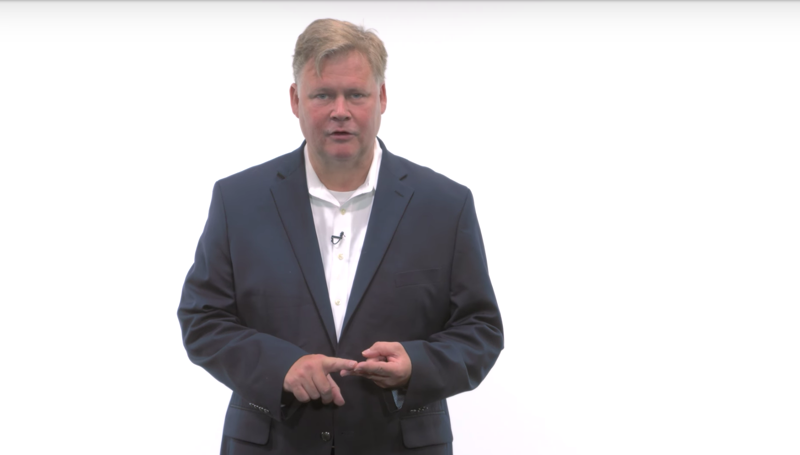• CAMP is one of the most significant ideas affecting finance in the last century.
• CAMP explains how the market impacts individual stock prices.
• CAMP provides a mathematical framework for hedge fund investing.
• It was developed by a number of researchers independently in the 1960s.
• William Sharpe, Harry Markowitz, and Merton Miller jointly received the Nobel Prize for this contribution in 1990.
• The CAPM led to the development of index funds and the belief that you can’t beat the market

Time: 00:00:36

# 02 - Definition of a portfolio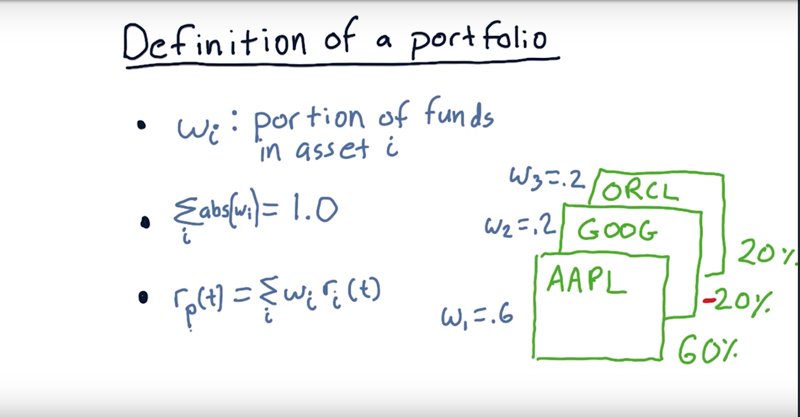Definition: A portfolio is a weighted set of assets.

• E.g.: a portfolio that’s got three different assets in it, Apple, Google, and Oracle. 60% in Apple, 20% in Google, and 20% in Oracle.
• 60%, 20% are the weights (wi)
• $\sum abs(w_i) = 100\%$ ( leveraged portfolio is a exception).
• returns on a portfolio: $R_p(t) = \sum_{i =1}^{n}(w_i * R_i(t))$, n = number of stocks in the portfolio.
• the equation allows short porsition and the return of it is opposite to the markect change.

ime: 00:02:05

# 03 - quiz Portfolio return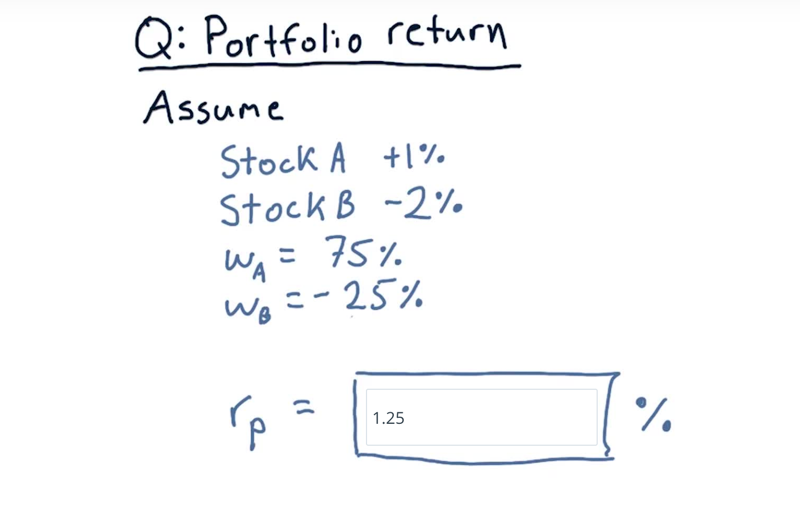weight stock return Position
75% A 1% Long
-25% B -2% Short

Given the portfolio and return of the stocks in it, calculate portfolio return

Solution: portfolio return = 75% * 1% + (-25%) * (-2%) = 1.25%

• the weight for Stock A is .75 and the return is 1%
• the weight for Stock B is negative 25% and return is 2% .
• since we shorted B and it went down, we got a positive component there, or .5.

Time: 00:00:39

# 04 - The market portfolio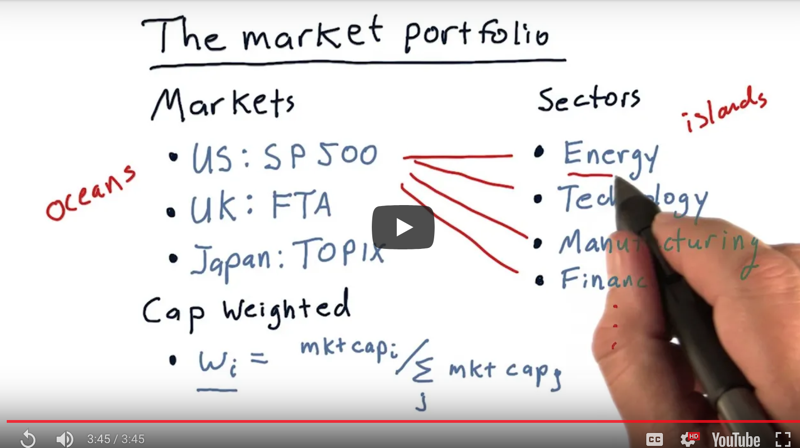The Market Portfolio usually referring to is an index that broadly covers a large set of stocks.

• e.g. S&P500. The S&P 500 represents the 500 largest companies that are traded
• The index changes each day according to the prices of all of its components.

The market portfolio is a combination of those stocks in a certain weighting.

• Cap Weighted: the weight of each stock in the index portfolio is usually set according to that stock’s market cap ($w_i = number\ of\ shares_i * price_i / \sum_{j=1}^{n}(market_Cap_j)$).

Sectors

• the US we typically break the market into ten different sectors, list four of them here. E.g.: Energy, technology, manufacturing, finance and so on.
• positive and negative news can affect each of these sectors individually without necessarily affecting the others.

So it’s not unusual to break up these large markets into individual sectors.

Note: some stocks have surprisingly large weightings (e.g. Apple and Exxon each are about 5% of the S&P 500) thus have a strong effect on what happens to this index.

Time: 00:03:46

# 05 - The CAPM equation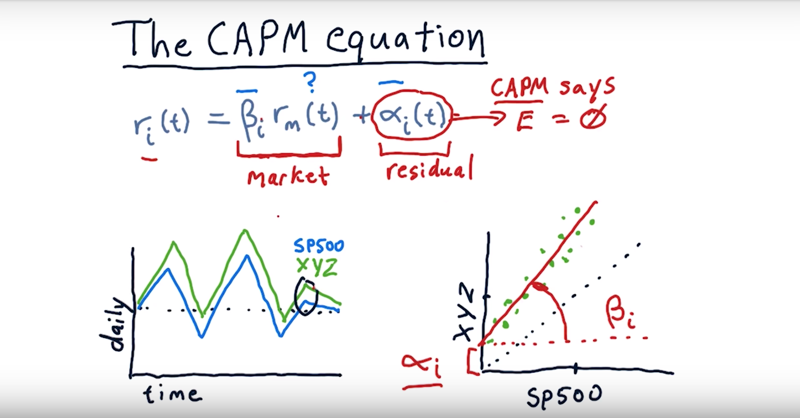The capital assets pricing model equation.

$r_i(t) = \beta_i * r_m(t) + \alpha_i(t)$

$r_i(t)$: The return of a particular stock, i, on a particular day, $\beta * r_m(t)$: the return for a particular stock due to the market.

• the market moving up or down strongly affects the change in price on every individual stock and $\beta$ is the extent of effects.
• every stock has it’s own $\beta$.
• $\alpha$ is called the residual which is the part that $\beta$ cannot predict.
• In the capital assets pricing model, the expectation for $\alpha$ is 0.

Where do we get this $\beta$ and this $\alpha$?

Depends on how the daily returns for a particular stock $r_i(t)$ related to the daily returns of the market $r_m(t)$.

• plot returns here for S&P 500 ($r_m(t)$.) against the daily return of an individual stock xyz ($r_i(t)$.) and fit a line to it. Then $\beta$ is the slop and $\alpha$ is the y-intercept.
• $\alpha$ and $\beta$ is based on historical data. in CAPM $\alpha$ are expect to be 0 (not always 0 in reality)

Time: 00:04:21

# 06 - quiz: Compare alpha and betaOf the two plots, which one has higher alpha and which one has a higher beta?

Solution: Correlated with SP500, ABC clearly has a greater slope than XYZ, therefore higher β. It also has a larger Y-intercept (α).

Time: 00:00:36

# 07 - CAPM vs active management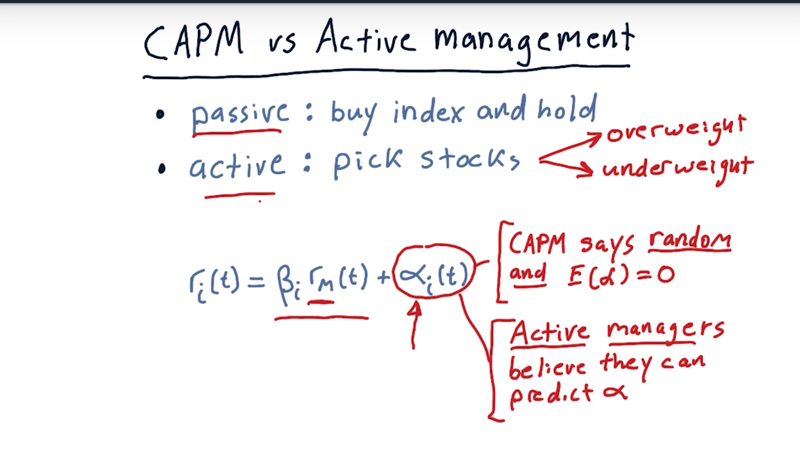• passive investing: essentially says that you should just buy an index portfolio, hold and let it grow.
• Active investing: Active portfolio managers don’t just buy the index portfolio, they pick individual stocks. s/he weights some stocks higher and others lowers, and select higher weighted ones for his/her portfolio

consider passive versus active in that context

• Both active managers and passive managers agree that the stock moves each day is most significantly influenced by the market $\beta * r_m(t)$.

• The CAPM the $\alpha$ is 1) random and 2) expected to be zero.
• Active managers believe they can predict $\alpha$ relative to the market.
• a passive investor believes the capital assets pricing model, Just buy an index and hold it.
• and active investor believes they or some else can find $\alpha$.

Time: 00:03:07

# 08 - CAPM for portfolios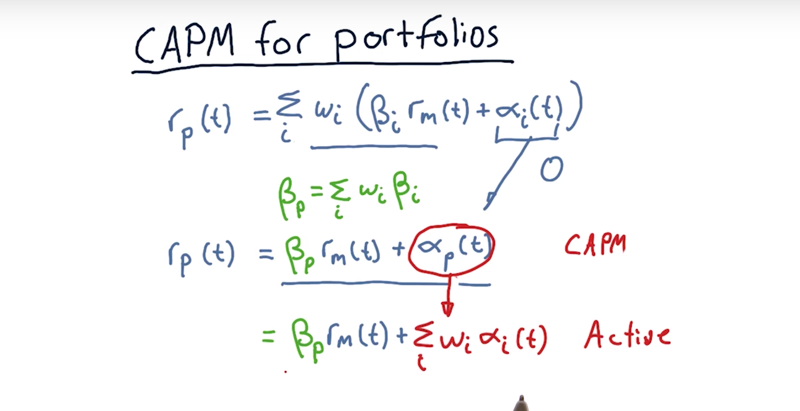the return for the entire portfolio

• compute this return for each individual stock$r_i(t)$, multiply it by the weight for that stock and then we take the sum across all the stocks.
• $r_p(t) = \sum(r_i(t) * w_i)$ (remember $r_i(t) = \beta_i * r_m(t) + \alpha_i(t))$

New way to calculate Beta

• $\beta_p = \sum(w_i(t) * \beta_i)$ and $\alpha_p = \sum(w_i(t) * \alpha_i(t))$ Thus,
• $r_p(t) = \beta_p * r_m(t) + \alpha_p$ for CAMP
• $r_p(t) = \beta_p * r_m(t) + \sum_i(w_i * alpha_i(t))$

Time: 00:02:04

# 9 - quiz: Implications of CAPM Quiz

Consider the implications of the CAPM, and implications in upward markets and downward markets.

If we’re in upward markets, do you want a larger beta or a smaller beta? And if we’re in downward markets, do you want a larger beta or a smaller beta?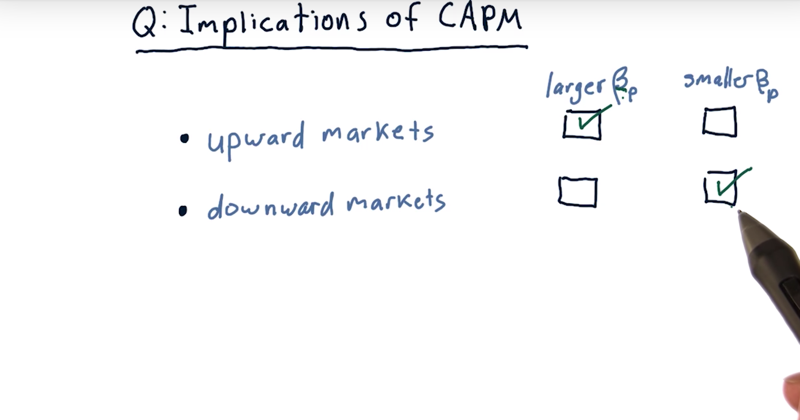solution: | market | stock | | ——– | —— | | Upward | larger | | Downward | smaller |

Time: 00:00:28

# 10 - Implications of CAPM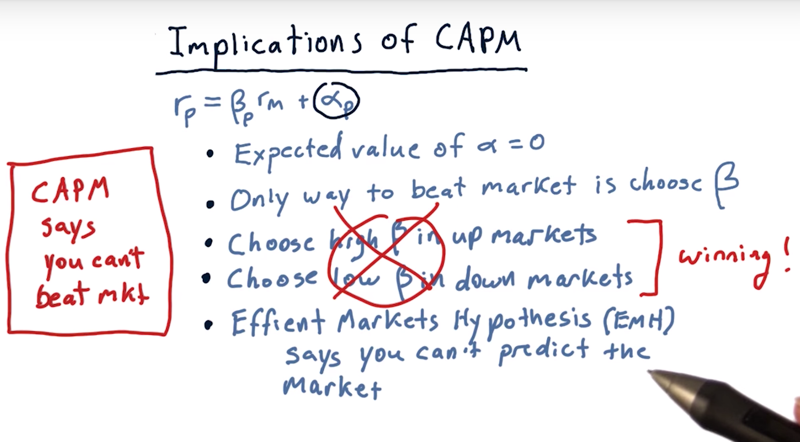• $\alpha$ is random, and the expected value of alpha is zero.
• the only way we can beat the market now is by cleverly choosing $\beta$, high $\beta$ in up markets and low $\beta$ in down markets.
• the efficient markets hypothesis says you can’t predict the market which means $\beta$ is not predictable.
• taken together, CAMP and the efficient markets hypothesis say that you can’t beat the market.

Now, we are on to ways that you can potentially beat the market

Time: 00:01:31

# 11 - Arbitrage Pricing Theory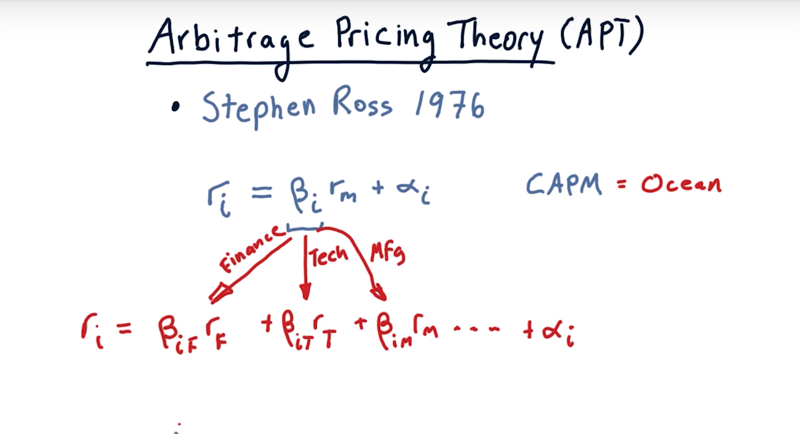• Arbitrage Pricing Theory was developed by Stephen Ross and first published in 1976.
• Dr. Ross though using single beta that represents a particular stock’s relationship to the market is not enough; Maybe multiple $\beta s$ against different sectors.
• And of course we still have our $\alpha$.
• $r_p(t) = \beta_{iF}*r_F + \beta_{iT} * r_T + \beta_{iM} * r_M + … … + \alpha_i$

Time: 00:01:38

Total Time: 00:22:12

2019-02-18 初稿